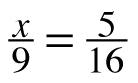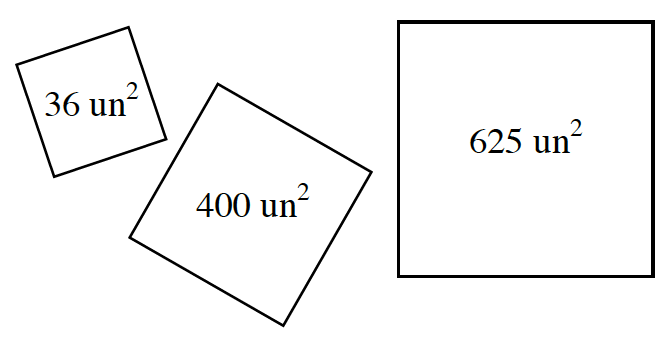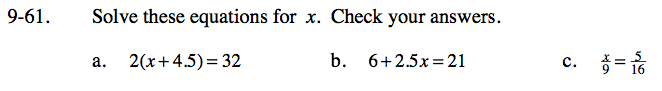### Home > CC3 > Chapter Ch9 > Lesson 9.2.1 > Problem9-61

9-61.
1. Solve these equations for x. Check your answers. Homework Help ✎

1. 2(x + 4.5) = 32

2. 6 + 2.5x = 21

3.Distribute.
2(x) + 2(4.5) = 32

Subtract 9 from both sides.

2x + 9 = 32
−9 −9
2x = 23

Divide by 2.

$\frac{2{\it x}}{2} = \frac{23}{2}$

x = 11.5

Isolate the variable.

See (a).

You could cross multiply.
16x = 5(9)

${\it x} = \frac{45}{16}$